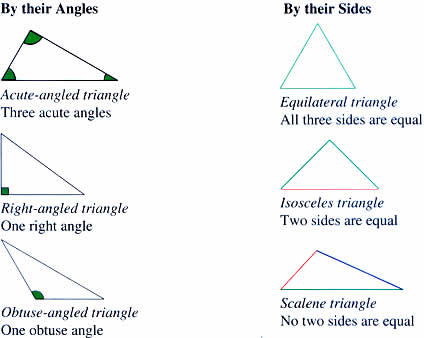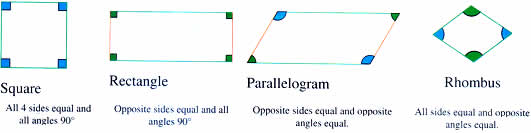Friday , September 18 2020# NCERT 5th Class (CBSE) Mathematics: Polygons And Circles

A polygon is a simple figure formed by connecting line segments. These line segments do not cross each other. Triangles and quadrilaterals (rectangles, squares, etc.) are examples of polygons.

## TRIANGLES

The word triangle means three angles. The given triangle has three angles ∠A, ∠B and ∠C. Points A, B and C are the vertices of triangle ABC. The sides of the triangle are AB, BC and CA.### Triangles can be classified: